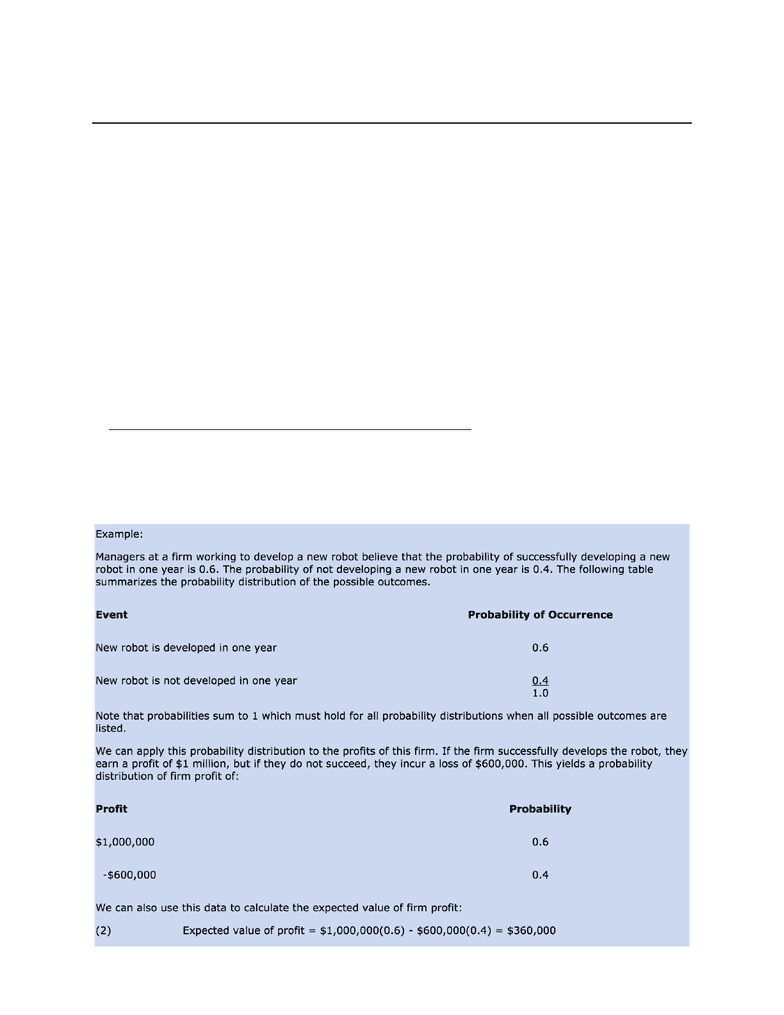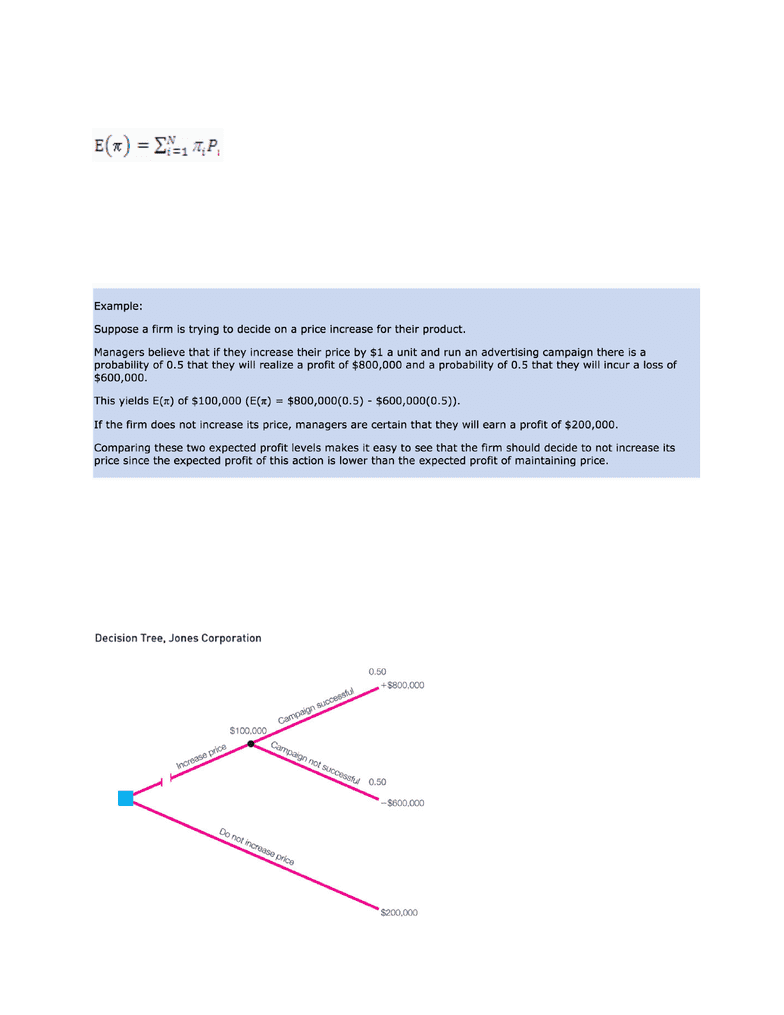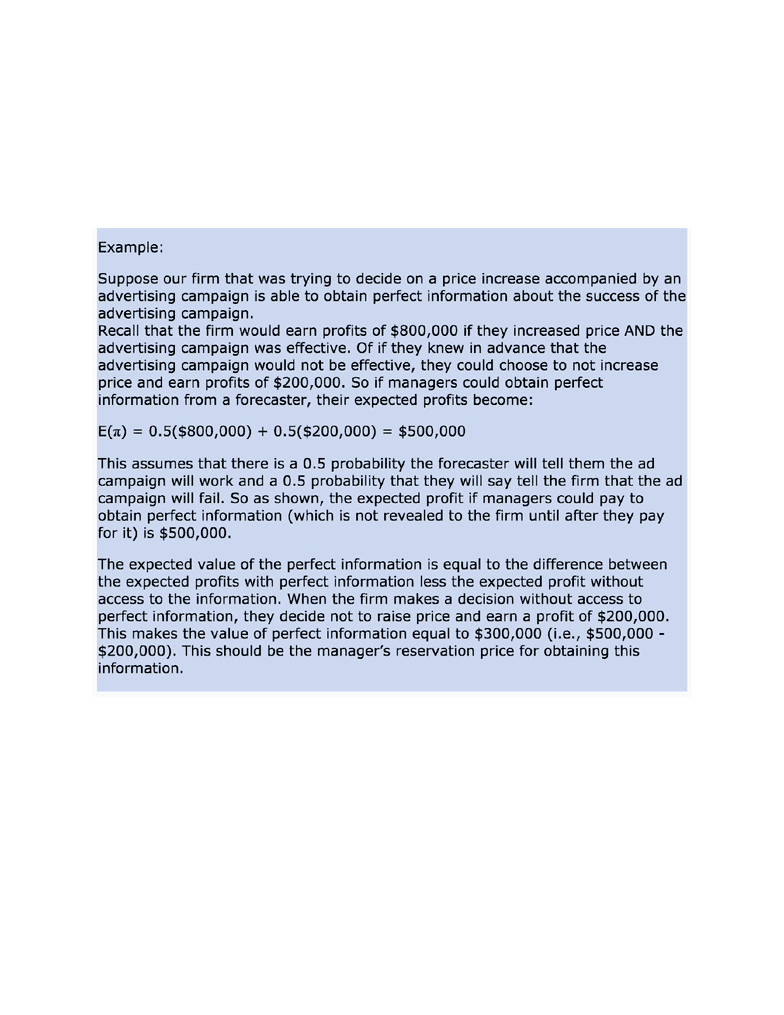Textbook Notes (270,000)
CA (160,000)
WLU (8,000)
EC (1,000)
EC260 (30)
Chapter 14

EC260 Chapter Notes - Chapter 14: Risk Premium, Utility, Risk Neutral

Department
Economics
Course Code
EC260
Professor
Olivia Ozlem Mesta
Chapter
14

This preview shows pages 1-3. to view the full 9 pages of the document.Chapter 14: Risk Analysis
Risk and Probability
-We define risk as a hazard or chance of experiencing a loss
-We define probability as the likelihood or chance that an event will take place
-If R is the number of times we repeat an experiment (i.e., rolling a single dice) and r is the
number of times the outcome A occurs, then the probability of A is defined as:
P(A) = r/R
-In rolling a single die, the probability of obtaining any of the six possible outcomes will be 1/6
— this is called the frequency definition of probability
-Since it is sometimes impossible or unrealistic to repeat an experiment over and over again,
managerial economists use a subjective definition of probability
-According to this definition, the probability of a certain outcome is the degree of confidence a
manager has that the outcome will occur
-The probabilities of all possible outcomes must sum to one
Probability Distributions and Expected Values
-When all possible outcomes are listed and a probability of occurrence is assigned to each
possible outcome, the resulting table is called a probability distribution

Only pages 1-3 are available for preview. Some parts have been intentionally blurred.-In general, we have the following expression for the expected value of profit:
-Where π is the profit associated with the ith possible outcome, Pi is the probability of the ith
outcome occurring, and N is the number of possible outcomes
Comparisons of Expected Profits
-A decision tree is a diagram managers can use to help them visualize what strategies to pursue
-At each point where the firm must make a decision, a series of branches represents the choices
they can make (a decision fork) — indicated by a small square
-At each point where chance or the actions affect the firm’s strategy, a series of branches
represents the possible outcomes (a chance fork)

Only pages 1-3 are available for preview. Some parts have been intentionally blurred.Expected Value of Perfect Information
-Imperfect or incomplete information is one source of uncertainty
-Sometimes managers can purchase information to remove some uncertainty
-The value of perfect information should be equal to the increase in expected profit resulting
-It is much harder to place a value on less than perfect information
A Utility Approach to Measuring Attitudes Towards Risk
-Up to this point, we have assumed that managers facing a risky decision will want to
maximize expected profit
-Some managers might not want to face the risk of a loss and might be inclined to choose a
less-profitable but certain outcome
-It is possible to construct a utility function for a manager that measures their attitude towards
risk